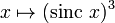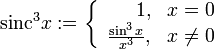# Sinc-cubed function

This article is about a particular function from a subset of the real numbers to the real numbers. Information about the function, including its domain, range, and key data relating to graphing, differentiation, and integration, is presented in the article.
View a complete list of particular functions on this wiki

## Definition

This function is defined as the composite of the cube function and the sinc function. Explicitly, it is given as:$x \mapsto (\operatorname{sinc} \ x)^3$

Alternatively, it is given as:$\operatorname{sinc}^3 x := \left\lbrace \begin{array}{rl} 1, & x = 0 \\ \frac{\sin^3x}{x^3}, & x \ne 0 \\\end{array}\right.$